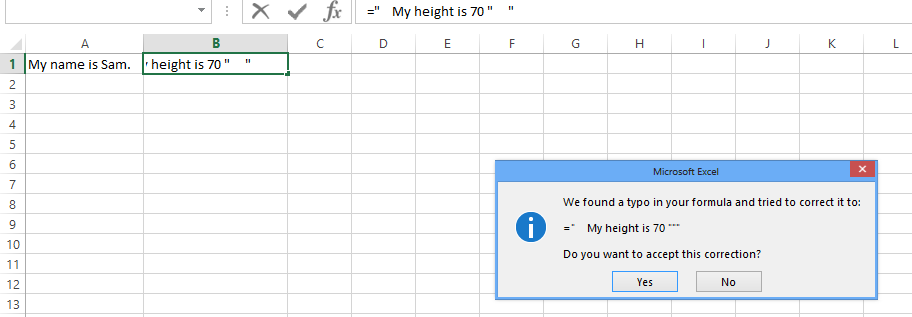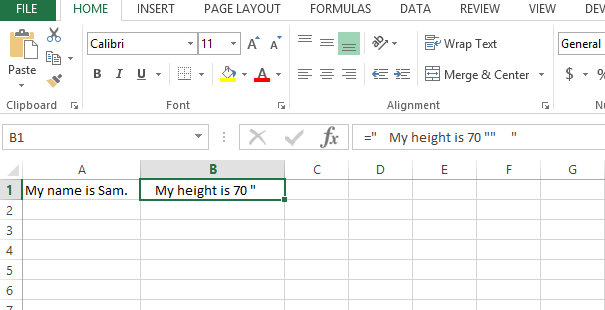### How To Quote A Quote In Excel Formula

In this Article I will guide you how to quote a quote in Excel formulas.

Excel formulas are used to do some simple calculations, to drive results using other cell values and sometimes we use some alphabetical strings in excel formulas which involve quotes : “.

E.g When the formula contain a string like :

a. My height is 70 “

b. My height is 70 inches.

Now statement b can easily be inserted in an excel formula like = “My height is 70 inches.”But for statement a we cannot write like: =” My height is 70 ” “It will return formula errors:So whenever we have quotes in a formula we have to understand that we have to escape the double quotes with double quotes to let Excel read it properly.

So the correct value: = ” My height is 70 “” “Another way to do this is using CHAR(34) for quotes:

Like we used quotes before quotes in previous example, here we are using char(34) instead of writing the quotes:

=” My height is 70 ” & CHAR(34)

And the result will be same: My height is 70 “## Template

```Further reading: